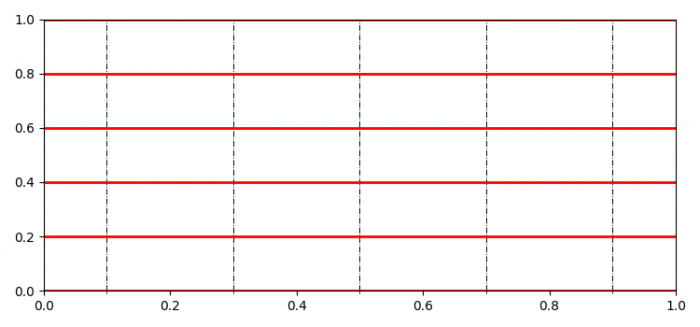# How to independently set horizontal and vertical, major and minor gridlines of a plot?

To set horizontal and vertical, major and minor grid lines of a plot, we can use grid() method.

## Steps

• Set the figure size and adjust the padding between and around the subplots.
• Create a figure and a set of subplots.
• Make horizontal grid lines for major ticks.
• Locate minor locator on the axes.
• Use grid() method to make minor grid lines.
• To display the figure, use show() method.

## Example

import matplotlib.pyplot as plt
from matplotlib.ticker import MultipleLocator

plt.rcParams["figure.figsize"] = [7.50, 3.50]
plt.rcParams["figure.autolayout"] = True

fig, ax = plt.subplots()

ax.yaxis.grid(which="major", color='r', linestyle='-', linewidth=2)
ml = MultipleLocator(0.10)

ax.xaxis.set_minor_locator(ml)
ax.xaxis.grid(which="minor", color='k', linestyle='-.', linewidth=0.7)

plt.show()

## Output